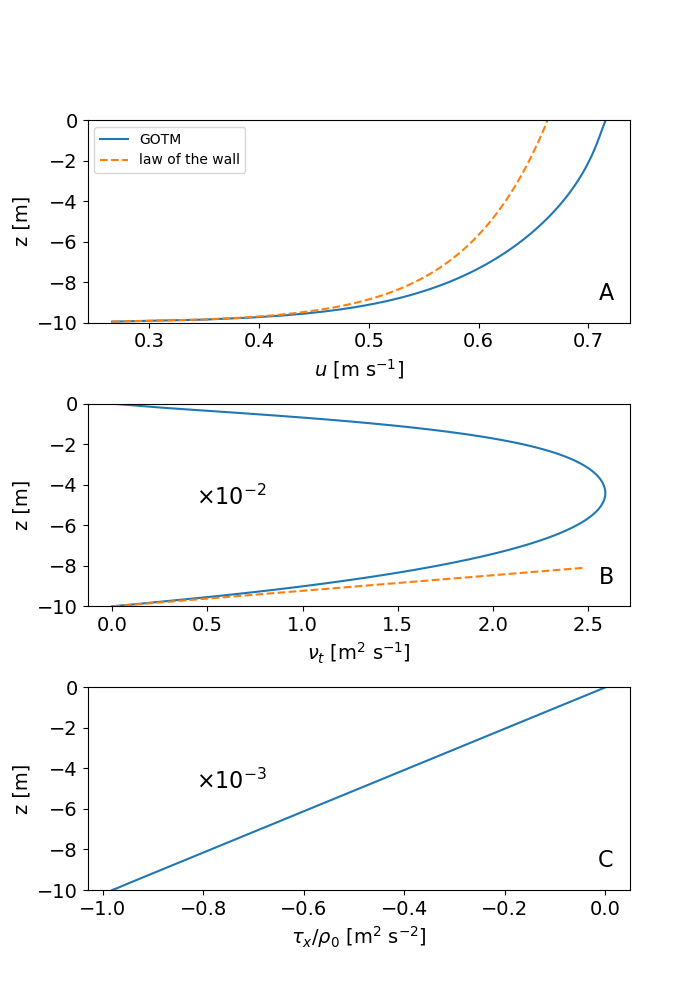# Channel flow

Similar to the idealized Couette scenario, also in this test case, an unstratified, non-rotating water column of 10 m thickness is investigated. Here, however, the flow is driven by a constant barotropic pressure gradient resulting from the tilt of the free surface. The surface stress is set to zero. This type of flow is often referred to as a turbulent open channel flow. The figure below shows the stationary solution approached at the end of the simulation. Different from the Couette scenario, the velocity profile shown in panel (a) exhibits only a single shear-layer at the bottom that converges toward the logarithmic wall layer close to the bottom. The turbulent diffusivity (panel b) is approximately symmetric but exhibits a slight vertical asymmetry due to the different boundary conditions at the surface and the bottom. Also this quantity converges toward the (linear) law-of-the-wall relation near the bottom. While the turbulent momentum flux is vertically constant in the Couette flow, for the open channel flow we find a linear variation. The vertical stress divergence is thus constant, and exactly balances the (constant) pressure gradient throughout the water column. The solution shown in the figure has been obtained with the k-ε model. Solutions for other two-equation models like the k-ω model or the Mellor-Yamada model look similar (you can easily check this in the scenario directory by modifying the input file gotm.yaml). Technical details for this test case are described in the GOTM documentation. We now also provide the python script plot_channel.py used to generate the figure for this GOTM scenario (you will find this plotting script also in the case directory).Profiles of (a) velocity, (b) turbulent diffusivity of momentum, and (c) turbulent momentum flux for the Channel scenario after stationarity has been reached (in red: law-of-the-wall solution)